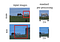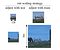# There’s a problem with image classifiers… and here’s the fix!

Writing this article after reading Fixing the train-test resolution discrepancy (Touvron et. al). I will include the relevant sections of the paper in brackets. I have also used images from the paper.

The current best practice for image preprocessing to train image classifiers looks a little something like this: (Section 1)

`train_transform = transforms.Compose([    transforms.RandomResizedCrop(224),    transforms.RandomHorizontalFlip(),    transforms.ToTensor(),    transforms.Normalize((0.5,0.5,0.5),(0.5,0.5,0.5))])test_transform = transforms.Compose([    transforms.Resize(256),    transforms.CenterCrop(224),    transforms.ToTensor(),    transforms.Normalize((0.5,0.5,0.5),(0.5,0.5,0.5))])`

We take different image preprocessings steps at train and test time. And here’s why. But this poses one problem: The size of objects in the images will be different at train and test time.The horse is visibly smaller at test time

This means the distribution of object sizes will be different in our train and test images. (Section 3.1) Our CNN struggles to learn scale invariant behavior.

The simple solution to this is to either reduce train-time resolution or increase test-time resolution:Reduce train-time resolution (Left) Increase test-time resolution (Right)

This has proven to improve accuracy. (Section 3.3)

However, using different resolutions at train and test time will affect the activation statistics of the CNN. (Section 3.2 Activation statistics) Keeping train-time resolution constant, increasing test-time resolution has shown to make activation maps (Figure 3)

• bigger in size,
• less sparse i.e more 0's,
• less spread out i.e more values below 2

This is a problem because the final classifier layers (linear & softmax) were trained for values of a different distribution.

To mitigate this, Touvron proposes fine-tuning the CNN with the catch of using images that have instead gone through the test-time preprocessing steps (Section 4.2). In PyTorch, this looks like:

`train_data = datasets.ImageFolder(train_path,                                  transform=test_transform # test DA                                 )trainloader_testDA = torch.utils.data.DataLoader(train_data, batch_size=bs, sampler=train_sampler)`

## Experiment results

The Fine Tuning column shows how fine tuning was conducted for experiment trials in the respective rows. A tick beneath Classifier (linear & softmax) and Batch-norm (immediate layer before classifier) represents if the respectives layers were fine-tuned (rest are frozen). Test DA (a.k.a train data augmentation) means test-time image preprocessing steps were taken for the fine tuning.

## Observations

The effect of using test-time image preprocessing for fine tuning does not have a significant impact on accuracy. It is only noticeable in trials where there’s a big difference in train-time and test-time resolutions. (Section 5.1 Ablation Study)

This table can act as a sort of rubric as to how we may pick our train-time and test-time resolutions to optimise training time, inference time or accuracy. For example, we can see that for a test-time resolution fixed at 224, we can lower the train-time resolution from 224 to 128 without sacrificing accuracy (Both 77.1%) thereby increasing training speeds.

## New state-of the-art!

This method also works with larger networks! Used with the ResNeXt-101 32x48d, this model is the new state-of-the-art with a 86.4% Top-1 accuracy. (Section 5.2).

## Closing remarks

Touvron’s method has improved the accuracy of image classifiers by “fixing” our problem of different object sizes. Let us take a moment to appreciate its name: FixRes.

I hope this has been informative and a good starting point for reading the paper for yourself.